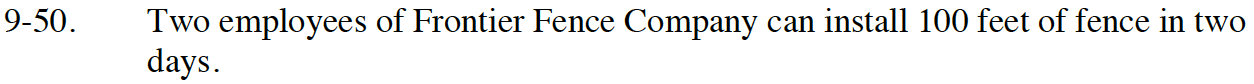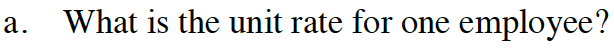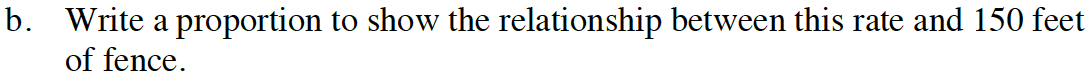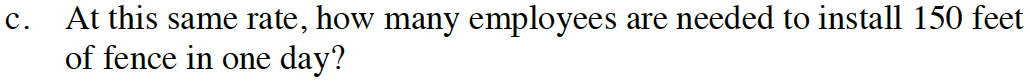Home > CC2MN > Chapter cc29 > Lesson cc29.1.3 > Problem9-50

9-50.
1. Two employees of Frontier Fence Company can install 100 feet of fence in two days. Homework Help ✎

1. What is the unit rate for one employee?

2. Write a proportion to show the relationship between this rate and 150 feet of fence.

3. At this same rate, how many employees are needed to install 150 feet of fence in one day?Create an expression by setting up a fraction with the given information.

$\text{rate}=\frac{\text{fencing per day}}{\text{number of people}}=\frac{\frac{100}{2}}{2}$

25 feet of fence per day per employeeUse the ratio from part (a) to help set up the proportion.Solve for the unknown in the proportion from part (b).

6 employees